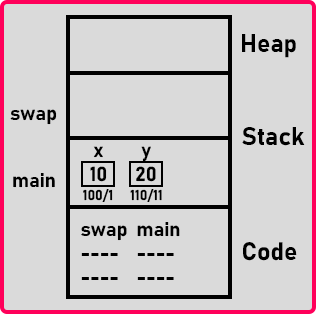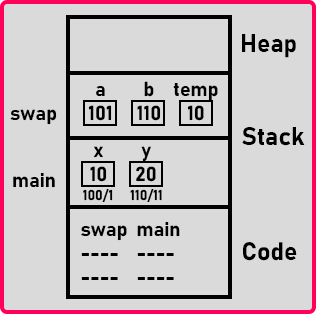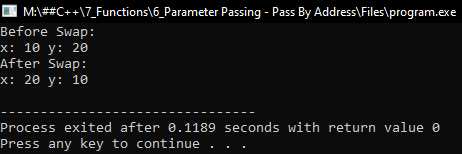# Parameter Pass by Address in C++

## Parameter Pass by Address in C++ with Examples:

In this article, I am going to discuss Parameter Pass by Address in C++ Language with Examples. Please read our previous article, where we discussed Parameter Pass by Value in C++ with Examples.

##### Parameter Pass by Address Mechanism in C++:

We have already seen the call by value or Pass by Value method in our previous article. In this article, we will discuss Call by Address or you can say Pass By Address.

void swap(int *a, int *b){
int temp;
temp = *a;
*a = *b;
*b = temp;
}
int main(){
int x = 10, y = 20;
swap(&x, &y);
cout << x << ” ” << y;
}

Here we have written the same code which we used in our previous article. But here we made some modifications to make the code for calling by address. Inside the main function, we have x and y variables and on the next line, we have called swap function. Now it will not send a value, it will send the addresses of variables x and y to swap function.

Then who can take addresses? We know well the variables who store addresses. So, pointers variables are used for storing addresses. In the ‘swap’ function, we made ‘a’ and ‘b’ as pointers by prefix with ‘*’. Now the formal parameters are pointers and actual parameters are addresses of variables.

Inside the swap function, if we want to swap the values of the variables then we have to write ‘*’ then _variable_name i.e. ‘*a’ that is dereferencing the pointers otherwise variable without ‘*’ will be the address, not the value. So, this is the code for the call-by address. This time ‘swap’ function will swap the values of ‘x’ and ‘y’ as it is called by address. This swap function can access actual parameters and it can modify them.

##### Memory Representation of Pass by Address in C++

Now let us see the memory representation of this swap program. The main function is the entry point so first the variables ‘x’ and ‘y’ are created. So ‘x’ and ‘y’ are created inside the stack memory. Let us say that the address of ‘x’ and ‘y’ are 100 and 110 as shown in the below image.Next, the ‘swap’ function is called. Address of ‘x’ and ‘y’ that is 100 and 110 will be sent to the ‘swap’ function. So, the activation record for swap is also created. For better understanding, please have a look at the below image.The swap function will have the variables ‘a’ and ‘b’ and these two variables are holding the address of ‘x’ and ‘y’. Here, we also created one temp variable. Next, we assign ‘temp = *a’, and temp will hold the value of ‘x’. The ‘*b = *a’ means value of a will be copied in ‘b’ i.e. ‘value of ‘x’ will be copied in ‘y’.

You can see that the ‘swap’ function is accessing the variables of the main function. We know that one function cannot access the variables of another function but it is possible through pointers. So, this is called by address mechanism. So, a pointer gives a pointer to a function to access the parameters of a calling function.

The ‘swap’ function is accessing ‘x’ and ‘y’. At end of this function, the value in ‘x’ will be 20, and the value in ‘y’ will be 10. So, their values have changed. Who has changed these values? ‘swap’ function. This is call by address.

If you want any function to modify the actual parameters then you can go for the call by address mechanism and one more thing it is not necessary that both the variables must be call by address only. One variable call by value, one variable call by address, or one variable call by reference. It all depends on the programmer’s requirement. Now let us see the complete program.

##### Parameter Pass by Address Program using C++:
```#include <iostream>
using namespace std;

void Swap(int *a, int *b)
{
int temp;
temp = *a;
*a = *b;
*b = temp;
}

int main()
{
int x = 10, y = 20;
cout << "Before Swap:\n";
cout << "x: " << x << " y: " << y << endl;
Swap (&x, &y);
cout << "After Swap:\n";
cout << "x: " << x << " y: " << y << endl;
}
```
###### Output: